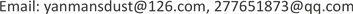1. 引言

2. 三种质量图2.1. 伪相干图

| z m , n | = ( ∑ cos ψ i , j ) 2 + ( ∑ sin ψ i , j ) 2 k 2 (1)

2.2. 相位导数方差图

| r m , n | = ∑ ( Δ i , j x − Δ ¯ m , n x ) 2 + ∑ ( Δ i , j y − Δ ¯ m , n y ) 2 k 2 (2)

2.3. 最大相位梯度图

| q m , n | = max { max { | Δ i , j x | } , max { | Δ i , j y | } } (3)

3. 卡尔曼滤波相位解缠基本原理

y ( k ) = [ Re { z ( k ) a ( k ) } Im { z ( k ) a ( k ) } ] ≜ [ cos ( φ ( k ) ) sin ( φ ( k ) ) ] + [ v 1 ( k ) v 2 ( k ) ] = h ( φ ( k ) ) + v ( k ) (4)

E { v ( k ) } = 0 ; E { v ( k ) v ( j ) T } = d i a g i = 1 , 2 [ 1 2 | γ | 2 − 1 2 ] δ ( k , j ) (5)

{ x ( k + 1 ) = A x ( k ) + u ( k ) u ( k ) = u ^ ( k ) + w ( k ) (6)

{ x ^ k + 1 , k = A x ^ k , k + u ^ k , k P k + 1 , k = A P k , k A T + Q k , k x ^ k + 1 , k + 1 = x ^ k + 1 , k + J k + 1 r k + 1 , k + 1 P k + 1 , k + 1 = ( I − J k + 1 C k + 1 , k ) P k + 1 , k J k + 1 = P k + 1 , k C k + 1 , k T ( C k + 1 , k P k + 1 , k C k + 1 , k T + R k + 1 , k + 1 ) − 1 r k + 1 , k + 1 = y k + 1 , k + 1 − C k + 1 , k x ^ k + 1 , k C k + 1 , k = [ − sin ( x ^ k + 1 , k ) , cos ( x ^ k + 1 , k ) ] T (7)

4. 实验结果与分析4.1. 地形陡峭条件下的实验结果与分析

ε = 1 M N ∑ i = 0 M − 2 ∑ j = 0 N − 1 ω i , j x | ϕ i + 1 , j − ϕ i , j − Δ i , j x | p + 1 M N ∑ i = 0 M − 1 ∑ j = 0 N − 2 ω i , j y | ϕ i , j + 1 − ϕ i , j − Δ i , j x | p (8)

Three quality maps phase unwrapping results’ number of discontinuous points and ε valu

4.2. 地形平坦条件下的实验结果与分析

Three quality maps phase unwrapping results’ number of discontinuous points and ε valu

5. 结论